imresize

Description

example

B = imresize(A,scale) returns image B that is scale times the size of image A. The input image A can be a grayscale, RGB, binary, or categorical image.

If A has more than two dimensions, then imresize only resizes the first two dimensions. If scale is between 0 and 1, then B is smaller than A. If scale is greater than 1, then B is larger than A. By default, imresize uses bicubic interpolation.

example

B = imresize(A,[numrows numcols]) returns image B that has the number of rows and columns specified by the two-element vector [numrows numcols].

example

[Y,newmap] = imresize(X,map,___) resizes the indexed image X where map is the colormap associated with the image.

example

___ = imresize(___,method) specifies the interpolation method used.

___ = imresize(___,Name,Value) returns the resized image where name-value arguments control various aspects of the resizing operation. Specify name-value arguments after all other input arguments.

Examples

collapse all

Shrink the image by a factor of two.

J = imresize(I, 0.5);

Display the original image and the resized image.

figure, imshow(I), figure, imshow(J)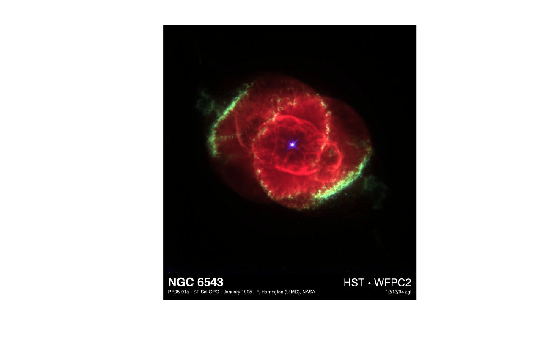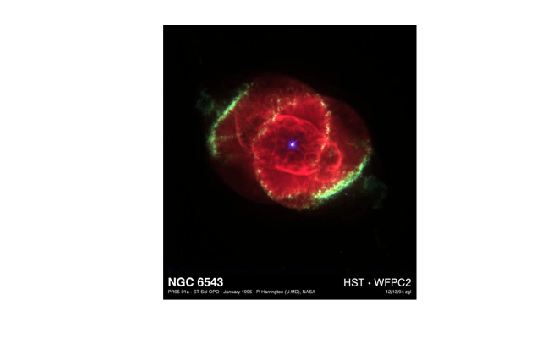Load an image into the workspace.

Shrink the image to 40% of the original size using nearest-neighbor interpolation. This is the fastest method, but it has the lowest quality.

J = imresize(I,0.4,'nearest');

Display the original image and the resized image.

imshow(I)
title('Original Image')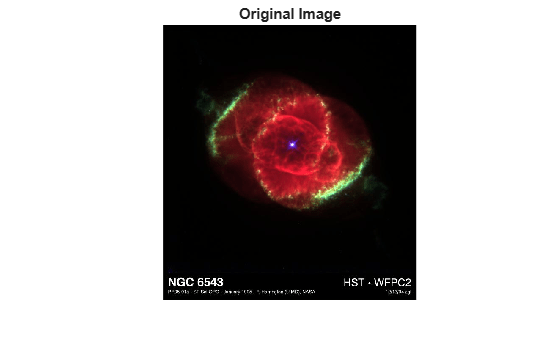imshow(J)
title('Resized Image Using Nearest Neighbor Interpolation')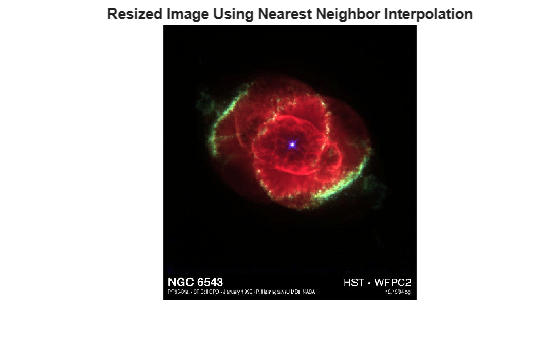Read an RGB image into the workspace.

Resize the RGB image to have 64 rows. imresize calculates the number of columns automatically.

RGB2 = imresize(RGB,[64 NaN]);

Get the size of the resized image.

sz = size(RGB2)
sz = 1×3

64    86     3

Display the original image and the resized image.

imshow(RGB)
title("Original Image")imshow(RGB2)
title("Resized Image with 64 Rows")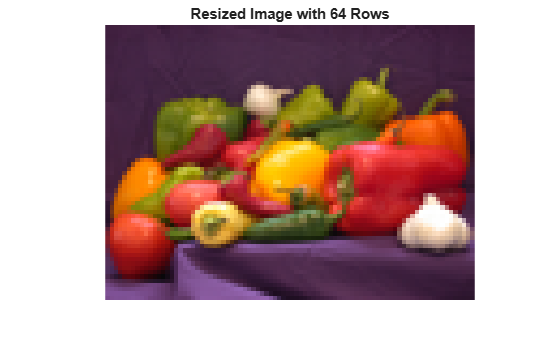Read an indexed image into the workspace.

Increase the size of the indexed image by 50%.

[Y,newmap] = imresize(X,map,1.5);

Display the original image and the resized image.

imshow(X,map)
title("Original Indexed Image and Colormap")imshow(Y,newmap)
title("Resized Image and Optimized Colormap")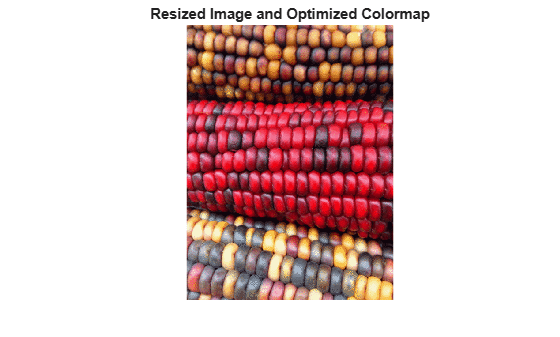Input Arguments

collapse all

Image to be resized, specified as a numeric array, logical array, or categorical array of any dimension. Input must be nonsparse, and numeric input must be real.

Data Types: single | double | int8 | int16 | int32 | uint8 | uint16 | uint32 | logical | categorical

Resize factor, specified as a positive number. imresize applies the same scale factor to the row and column dimensions. To apply a different resize factor to each dimension, use the Scale name-value argument.

Data Types: single | double | int8 | int16 | int32 | int64 | uint8 | uint16 | uint32 | uint64

Row and column dimensions of output image, specified as a two-element vector of positive numbers. You can specify the value NaN for either numrows or numcols. In this case, imresize computes the number of rows or columns for that dimension automatically, preserving the aspect ratio of the image.

Data Types: single | double | int8 | int16 | int32 | int64 | uint8 | uint16 | uint32 | uint64

Indexed image to be resized, specified as a real, nonsparse numeric array of positive integers.

Data Types: double | uint8 | uint16

Colormap associated with indexed image X, specified as a c-by-3 numeric matrix with values in the range [0, 1]. Each row is a three-element RGB triplet that specifies the red, green, and blue components of a single color of the colormap.

Data Types: double

Interpolation method, specified as a character vector, string scalar, or two-element cell array.

When method is a character vector or string scalar, it identifies a particular method or named interpolation kernel, listed in the following table.

MethodDescription

"nearest"

Nearest-neighbor interpolation; the output pixel is assigned the value of the pixel that the point falls within. No other pixels are considered.

"bilinear"

Bilinear interpolation; the output pixel value is a weighted average of pixels in the nearest 2-by-2 neighborhood

"bicubic"

Bicubic interpolation; the output pixel value is a weighted average of pixels in the nearest 4-by-4 neighborhood

Note

Bicubic interpolation can produce pixel values outside the original range.

Interpolation KernelDescription
"box"Box-shaped kernel
"triangle"Triangular kernel (equivalent to "bilinear")
"cubic"Cubic kernel (equivalent to "bicubic")
"lanczos2"Lanczos-2 kernel
"lanczos3"Lanczos-3 kernel

When method is a two-element cell array, it defines a custom interpolation kernel. The cell array has the form {f,w}, where f is a function handle for a custom interpolation kernel and w is the width of the custom kernel. f(x) must be zero outside the interval -w/2 <= x < w/2. The function handle f can be called with a scalar or a vector input. For user-specified interpolation kernels, the output image can have some values slightly outside the range of pixel values in the input image.

Data Types: char | string | cell

Name-Value Arguments

Specify optional comma-separated pairs of Name,Value arguments. Name is the argument name and Value is the corresponding value. Name must appear inside quotes. You can specify several name and value pair arguments in any order as Name1,Value1,...,NameN,ValueN.

Example: 'Antialiasing',false does not perform antialiasing when shrinking an image

Perform antialiasing when shrinking an image, specified as the comma-separated pair consisting of 'Antialiasing' and true or false.

• If the interpolation method is "nearest", then the default value of 'Antialiasing' is false.

• If the interpolation method is "box" and the input image is categorical, then the default value of 'Antialiasing' is false.

• For all other interpolation methods, the default value is true.

Data Types: logical

Return optimized or original colormap for indexed image, specified as the comma-separated pair consisting of 'Colormap' and one of the following values.

ValueDescription
"original"The output colormap newmap is the same as the input colormap map.
"optimized"imresize returns a new optimized colormap.

The 'Colormap' argument is valid only when resizing indexed images.

Data Types: char | string

Perform color dithering, specified as the comma-separated pair consisting of 'Dither' and true or false. In dithering, you apply a form of noise to the image to randomize quantization error and prevent large-scale patterns.

The 'Dither' argument is valid only when resizing indexed images.

Data Types: logical

Interpolation method, specified as the comma-separated pair consisting of 'Method' and a character vector or two-element cell array. For details, see method.

Data Types: char | string | cell

Size of the output image, specified as the comma-separated pair consisting of 'OutputSize' and a two-element vector of positive numbers. For details, see [numrows numcols].

Data Types: single | double | int8 | int16 | int32 | int64 | uint8 | uint16 | uint32 | uint64

Resize scale factor, specified as the comma-separated pair consisting of 'Scale' and a positive number or two-element vector of positive numbers. If you specify a scalar, then imresize applies the same scale factor to the row and column dimensions. If you specify a two-element vector, then imresize applies a different scale value to each dimension.

Data Types: single | double | int8 | int16 | int32 | int64 | uint8 | uint16 | uint32 | uint64

Output Arguments

collapse all

Resized image, returned as a numeric, logical, or categorical array of the same data type as the input image, A.

Resized indexed image, returned as a numeric array of the same data type as the input indexed image, X.

Colormap of the resized indexed image Y, returned as an m-by-3 numeric matrix. By default, imresize returns a new, optimized colormap with the resized image. To return a colormap that is the same as the original colormap, use the 'Colormap' name-value argument.

Tips

• If the size of the output image is not an integer, then imresize does not use the scale specified. imresize uses ceil when calculating the output image size.